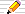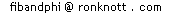This is an option extra page summarizing the method of Proof By Induction, applied to a particular Fibonacci number formula. We show how not to use the method also as well as the complete proof of the formula.

Theicon means there is a Things to do investigation at the end of the section.

# The Powers of Phi Formula to prove

The formula we will prove is
Phin = Fib(n+1) + Fib(n) phi
We assume the standard definition of Fib(n):
Fib(n+2)=Fib(n+1)+Fib(n) for all n>0;
Fib(0)=0 and Fib(1)=1
and for Phi and phi we use the definitions
 Phi = √5 + 1 phi = √5 – 12 2

## What is a Proof By Induction?

A proof by induction involves two steps:
1. Proving that IF the above formula is true for any particular value of n, let's say n=k, then it must automatically follow that it isrue for k+1 too.

Since (k+1) is another particular value, the same argument shows the formula is therefore true for k+2. "By induction" we can therefore reason that it will be true for all following values of n from k onwards.

Note that we assume the formula is true for n=k and, on that basis, show that it must inevitably be true for the next larger value k+1.
This assumption f(that the formula is true for n=k in particular) is called the Inductive Assumption. It is not the same as assuming the formula is true for all n, since we are only assuming it is true for one particular value of n, namely n=k.

The proof that "IF the formula is true for n=k THEN it must also be true for n=k+1 also" must be sufficiently general that it applies to all values of k, that is, it does not rely on specific values of k to work.

2. There must be a value of n for which the formula can be shown to be true.

This value will start off the "induction" process. So, for the formula to be true for all n we need the starting value to be n=1 or perhaps n=0.

## A Faulty Proof By Induction

Failure to observe these two conditions fully gives rise to faulty induction "proofs".
For instance, here is a "proof" that All cars are red. We show a faulty application of proof by induciton to the statement All sets of n cars are red cars. and show it is true for all values of n:
1. Suppose that we have a specific instance where it is true: n=k.
Our assumption is that ALL sets of k cars contain only red cars.
So let's take any other car and add it in to make a set of k+1 cars.
We need to show it is red too.
To show this new set contains only red cars means we have to show the new car is red too.
We do this by considering a subset of the new set formed by taking out any other car from the set, leaving a set of size n containing the original assumption that all sets of size k are of red cars then this set is too.
But that set contains the new car and so we have shown, on the basis if the assumption that the new car is red too.
2. My car is red so I can find a set of size 1 for which the statement is true.
So where has this "proof" gone wrong since clearly there are cars of other colours than red?
Well, although there is no fault in the reasoning of the inductive part of the argument (stage 1 above), the second part is not always true. Whilst my car is red, my neighbour's car is white, so it wouldn't work for him! We cannot verify the second condition, that the statement "All sets of 1 car are red cars" is always true.

So we have to be particularly careful as to what the statement is (about n) that we are trying to prove.

## Proving this Formula by Induction

First, assume it is true for n=k, that is that
Phik = Fib(k+1) + Fib(k) phi -- our starting assumption
and we want to show that
Phik+1 = Fib(k+2) + Fib(k+1) phi
Since the left hand sides differ by a factor of Phi, let's start by multiplying our assumed result by Phi on both sides:
 Phi × Phik = Phi × ( Fib(k+1) + Fib(k) phi ) Phik+1 = Fib(k+1) Phi + Fib(k) Phi phi Phik+1 = Fib(k+1) Phi + Fib(k) using Phi phi = 1 Phik+1 = Fib(k+1) (1 + phi) + Fib(k) since Phi = 1 + phi Phik+1 = Fib(k+1) + Fib(k) + Fib(k+1) phi by rearranging Phik+1 = Fib(k+2) +Fib(k+1) phi by the Fibonacci Rule
Notice that the above argument would work no matter what the value of k is.

Secondly, we need to find a starting value for n for which the formula is true.
When n=0, we have

Phi0 = Fib(0+1) + Fib(0) phi
which simplifies to
Ph0 = 1 + 0 phi = 1
which we know is true.

So the two parts of our proof by induction are now complete. Things to do© 2003 Dr Ron Knottcreated 26 November 2003